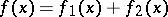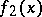# Function of bounded variation

(diff) ← Older revision | Latest revision (diff) | Newer revision → (diff)
A function whose variation is bounded (see Variation of a function). The concept of a function of bounded variation was introduced by C. Jordan  for functions of one real variable in connection with a generalization of the Dirichlet theorem on the convergence of Fourier series of piecewise-monotone functions (see Jordan criterion for the convergence of a Fourier series). A functiondefined on an intervalis a function of bounded variation if and only if it can be represented in the form, where(resp.,) is an increasing (resp., decreasing) function on(see Jordan decomposition of a function of bounded variation). Every function of bounded variation is bounded and can have at most countably many points of discontinuity, all of the first kind. A function of bounded variation can be represented as the sum of an absolutely-continuous function (see Absolute continuity), a singular function and a jump function (see Lebesgue decomposition of a function of bounded variation).